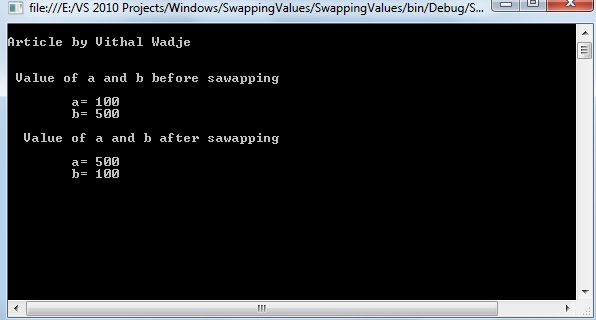Featured

# Swapping two Values Using C#

In this article we will learn  how to swap two values without creating a new storage location using  C#, this type of question might be asked by an interviewer in a .Net position related interview.
My intent for this article is to explain how to answer a question that is often asked in an interview, which is: Write a program to swap two values using C# without creating the new storage location. A candidate new to the interview can become confused because the first problem is the candidate does not even know which keyword to use for that, so how will he or she know how to write the program?
So considering the preceding requirement I have decided to write this article with the basics and especially focusing on the beginner, student and whoever might encounter this type of question in an interview.
What does swapping values mean?
When the values of two variables are exchanged at run time it is called swapping of the two values.
Example:
a=100;
b=500;
After swapping:
a=500;
b=100;
Conditions to swap values
The following re the conditions to swap values:
• The values of two variable are swapped with each other without creating a new storage location for the variables
• After the swapping of the values of the two variables then before swapped values are need to be remain in that variable.
Depending on the preceding condition we need to swap the values, then after that reading the preceding condition, you are thinking, how is it possible, but it is possible by using the ref keyword of C# that does not create the new storage location for the values.
Refer to my following article to learn the details about the ref parameter:
Next I will explain how to do it step-by-step, as in the following:
1. Open Visual Studio from "Start" - - "All programs" -- "Microsoft Visual Studio".
2. Then go to to "File" -> "New" -> "Project..." then select "Visual C#" -> "Windows" -> "Console application".
3. Then specify the name, "SwappingNum", or whatever name you wish and the location of the project and click on the "OK" button. The new project is created.
Now create the following ref parameter method:
```static void SwapNum(ref int x, ref int y)
{

int tempswap = x;
x = y;
y =tempswap;
}
```
In the preceding method we are using the ref parameter to take the reference of values from other variables that do not create any new storage location.
Now create the calling method as:
```static void Main(string[] args)
{
int a = 100;
int b = 500;

Console.WriteLine( "Value of a and b before sawapping");
Console.WriteLine();
Console.WriteLine("a=" + " " + a);
Console.WriteLine( "b=" + " " + b);
SwapNum(ref a, ref b);
Console.WriteLine();
Console.WriteLine("Value of a and b after sawapping");
Console.WriteLine();
Console.WriteLine("a=" + " " + a);
Console.WriteLine("b=" + " " + b);

}
```
In the preceding main calling method we are calling the SwapNum method and passing the reference of  a and b variable values to the method, now the entire code will like as follows:
```using System;

namespace SwappingValues
{
class Program
{
static void SwapNum(ref int x, ref int y)
{

x = y;
y = x;

}
static void Main(string[] args)
{
int a = 100;
int b = 500;

Console.WriteLine( "Value of a and b before sawapping");
Console.WriteLine();
Console.WriteLine("a=" + " " + a);
Console.WriteLine( "b=" + " " + b);
SwapNum(ref a, ref b);
Console.WriteLine();
Console.WriteLine("Value of a and b after sawapping");
Console.WriteLine();
Console.WriteLine("a=" + " " + a);
Console.WriteLine("b=" + " " + b);

}
}
}
```
Now run the program; the output will be as:From the preceding program its clear that we have swapped the values of two variables without creating a new storage location and also the original values of  a and b remain as they were, in other words not changed.
Summary